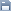Leetcode
2020.04.25 15:54

# 38. Count and Say

조회 수 107 추천 수 0 댓글 0
?

#### 단축키

Prev이전 문서

Next다음 문서

크게 작게 위로 아래로 댓글로 가기 인쇄
?

#### 단축키

Prev이전 문서

Next다음 문서

크게 작게 위로 아래로 댓글로 가기 인쇄

The count-and-say sequence is the sequence of integers with the first five terms as following:

```1.     1
2.     11
3.     21
4.     1211
5.     111221
```

`1` is read off as `"one 1"` or `11`.
`11` is read off as `"two 1s"` or `21`.
`21` is read off as `"one 2`, then `one 1"` or `1211`.

Given an integer n where 1 ≤ n ≤ 30, generate the nth term of the count-and-say sequence. You can do so recursively, in other words from the previous member read off the digits, counting the number of digits in groups of the same digit.

Note: Each term of the sequence of integers will be represented as a string.

Example 1:

```Input: 1
Output: "1"
Explanation: This is the base case.
```

Example 2:

```Input: 4
Output: "1211"
Explanation: For n = 3 the term was "21" in which we have two groups "2" and "1", "2" can be read as "12" which means frequency = 1 and value = 2, the same way "1" is read as "11", so the answer is the concatenation of "12" and "11" which is "1211".```

```class Solution {
public String countAndSay(int n) {
if(n <= 0){
return null;
}

String result = "1";

for(int i=1; i<n; i++){
//String str = "";
StringBuilder sb = new StringBuilder();
int count = 1;

for(int j=1; j<result.length(); j++){
if(result.charAt(j) == result.charAt(j - 1)){
count++;
}else{
//str = str + (count + "" + (result.charAt(j - 1)));
sb.append(count);
sb.append(result.charAt(j - 1));
count = 1;
}
}

//str = str + (count + "" + result.charAt(result.length() - 1));
//result = str;
sb.append(count);
sb.append(result.charAt(result.length() - 1));
result = sb.toString();
}

return result;
}
}```

?

 제목+내용제목내용댓글이름닉네임아이디태그
List of Articles
번호 분류 제목 글쓴이 날짜 조회 수
74 Leetcode 692. Top K Frequent Words 2020.04.15 93
73 Leetcode 2. Add Two Numbers 2020.04.14 96
72 Leetcode 731. My Calendar II 2020.04.15 100
71 Leetcode 75. Sort Colors 2020.04.14 101
70 Leetcode 347. Top K Frequent Elements 2020.04.14 102
69 Leetcode 20. Valid Parentheses 2020.04.25 102
68 Leetcode 430. Flatten a Multilevel Doubly Linked List2020.04.06 104
67 Leetcode 23. Merge k Sorted Lists 2020.04.14 104
66 Leetcode 729. My Calendar I 2020.04.15 104
65 Leetcode 253. Meeting Rooms II 2020.04.15 104
64 Leetcode 852. Peak Index in a Mountain Array 2020.04.28 104
63 Leetcode 7. Reverse Integer 2020.04.28 104
62 Leetcode 62. Unique Paths2020.04.09 105
61 Leetcode 350. Intersection of Two Arrays II 2020.04.28 106
60 Leetcode 946. Validate Stack Sequences 2020.04.08 107
59 Leetcode 973. K Closest Points to Origin 2020.04.15 107
58 Leetcode 443. String Compression 2020.04.25 107
» Leetcode 38. Count and Say 2020.04.25 107
56 Leetcode 53. Maximum Subarray 2020.04.28 110
55 Leetcode 937. Reorder Data in Log Files 2020.04.25 111
Board Pagination Prev 1 2 3 4 Next
/ 4